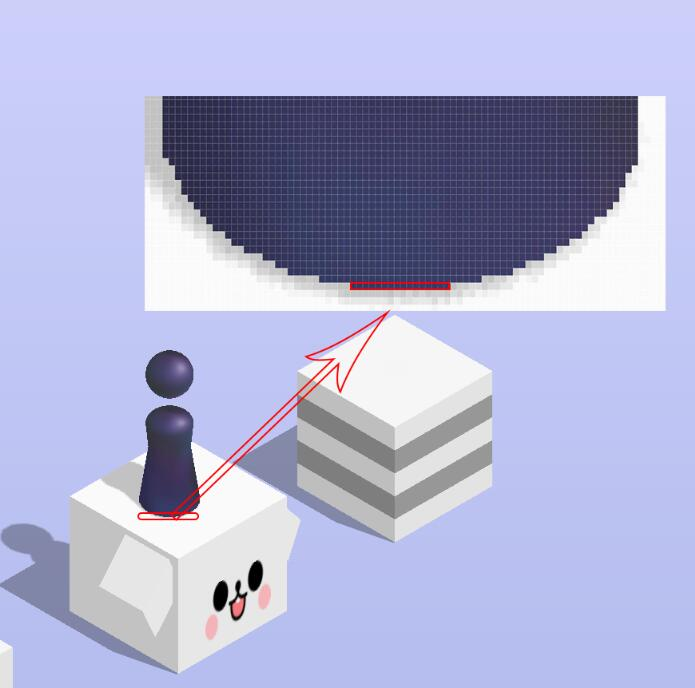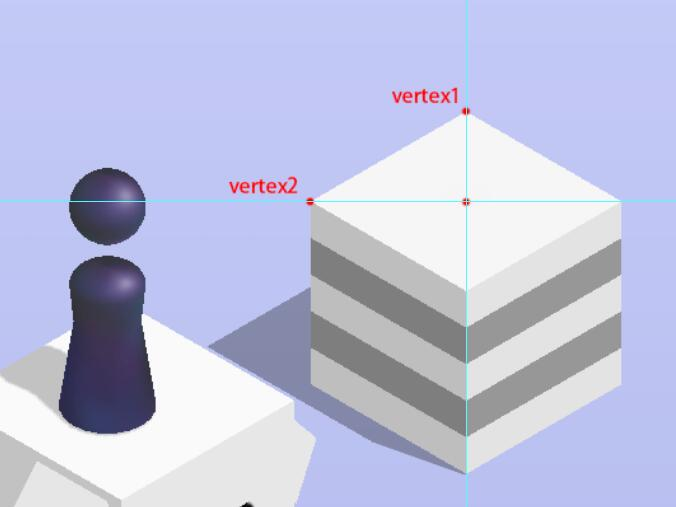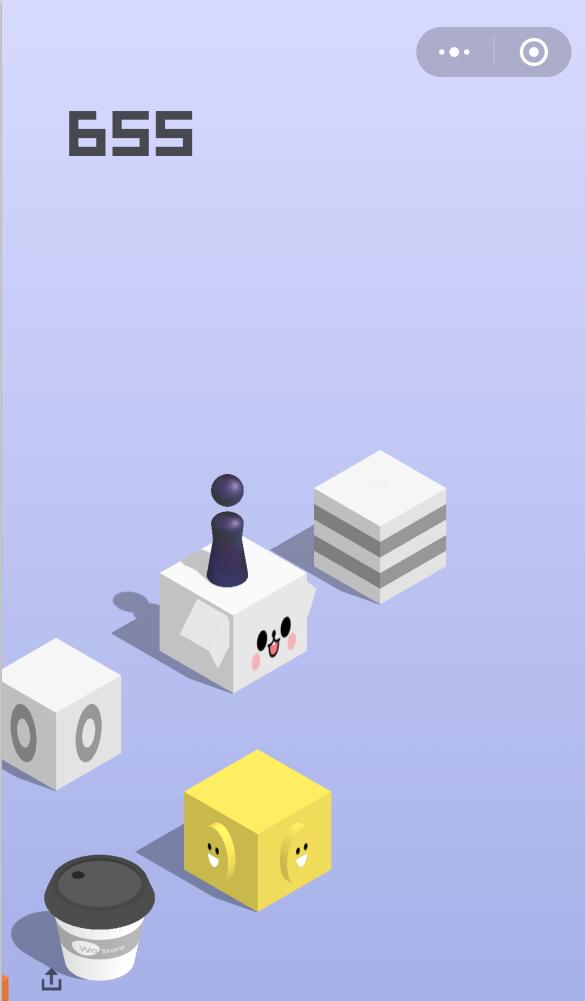### Python编程语言

Python 是一种面向对象、解释型计算机程序设计语言，由Guido van Rossum于1989年底发明，第一个公开发行版发行于1991年。Python语法简洁而清晰，具有丰富和强大的类库。它常被昵称为胶水语言，它能够把用其他语言制作的各种模块（尤其是C/C++）很轻松地联结在一起。

1. 每次跳跃之前，截取一下手机屏幕，并将截图保存到本地电脑中；
2. 计算截图中人偶的位置与将要跳至的台面中心的距离dd；
3. 将以上距离dd换算成相应的触摸时间ss；
4. 发送模拟触摸的命令至手机，触摸时间为以上时间ss；

`adb devices`

``````

````````````
s=d∗1.35
s=d∗1.35``````

``````
adb shell input swipe 0 0 0 0 1000````````````
import math
import os
import tempfile
import time
from functools import reduce
from PIL import Image
BACKGROUND_POS = (40, 500)
DISTANCE_TO_TIME_RATIO = 1.35
SCREENSHOT_PATH = tempfile.gettempdir() + "/screenshot.png"
def calculate_jump_distance():
im = Image.open(SCREENSHOT_PATH)
background_rgb = im.getpixel(BACKGROUND_POS)
role_pos_list = None
vertex1_pos = None
block_background_rgb = None
vertex2_pos = None
role_line_flag = True
for y in range(BACKGROUND_POS, im.height):
if role_pos_list and role_line_flag:
break
role_line_flag = True
vertex2_line_flag = True
for x in range(BACKGROUND_POS, im.width):
current_rgb = im.getpixel((x, y))
next_rgb = im.getpixel((x + 1, y)) if x + 1 < im.width else (0, 0, 0)
# 识别顶点1
if x > BACKGROUND_POS and y > BACKGROUND_POS and not vertex1_pos \
and not is_similar(background_rgb, current_rgb) and is_similar(current_rgb, next_rgb):
vertex1_pos = (x, y)
block_background_rgb = current_rgb
# 识别顶点2
if block_background_rgb and vertex2_line_flag and is_similar(current_rgb, block_background_rgb, 5):
vertex2_line_flag = False
if vertex2_pos:
if x < vertex2_pos and vertex2_pos - x < 20 and y - vertex2_pos < 20:
vertex2_pos = (x, y)
else:
vertex2_pos = (x, y)
# 识别小人
if is_part_of_role(current_rgb):
if role_line_flag:
role_pos_list = []
role_line_flag = False
role_pos_list.append((x, y))
if len(role_pos_list) == 0:
raise Exception('无法识别小人位置！！！')
pos_sum = reduce((lambda o1, o2: (o1 + o2, o1 + o2)), role_pos_list)
role_pos = (int(pos_sum / len(role_pos_list)), int(pos_sum / len(role_pos_list)))
destination_pos = (vertex1_pos, vertex2_pos)
return int(linear_distance(role_pos, destination_pos))
def is_part_of_role(rgb):
return 53 < rgb < 59 and 57 < rgb < 61 and 95 < rgb < 103
def linear_distance(xy1, xy2):
return math.sqrt(pow(xy1 - xy2, 2) + pow(xy1 - xy2, 2))
def is_similar(rgb1, rgb2, degree=10):
return abs(rgb1 - rgb2) <= degree and abs(rgb1 - rgb2) <= degree and abs(rgb1 - rgb2) <= degree
def screenshot():
os.system("adb pull /mnt/sdcard/screencap.png {} >> {}/jump.out".format(SCREENSHOT_PATH, tempfile.gettempdir()))
def jump(touch_time):
os.system("adb shell input swipe 0 0 0 0 {}".format(touch_time))
def distance2time(distance):
return int(distance * DISTANCE_TO_TIME_RATIO)
if __name__ == '__main__':
count = 1
while True:
screenshot()
distance = calculate_jump_distance()
touch_time = distance2time(distance)
jump(touch_time)
print("#{}: distance={}, time={}".format(count, distance, touch_time))
count += 1
time.sleep(1)``````

100行python代码实现跳一跳辅助程序
50行python代码实现的贪吃蛇小游戏

21行Python代码实现拼写检查器
200 行python 代码实现 2048 游戏
1 行 Python 代码快速实现 FTP 服务器